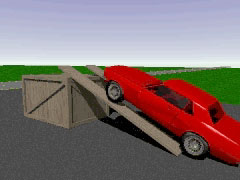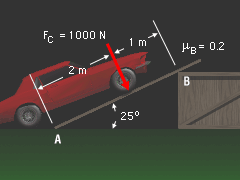Ch 9. Friction Multimedia Engineering Statics Friction I Friction II
 Chapter 1. Basics 2. Vectors 3. Forces 4. Moments 5. Rigid Bodies 6. Structures 7. Centroids/Inertia 8. Internal Loads 9. Friction 10. Work & Energy Appendix Basic Math Units Sections Search eBooks Dynamics Fluids Math Mechanics Statics Thermodynamics Author(s): Kurt Gramoll ©Kurt GramollSTATICS - CASE STUDY IntroductionProblem Graphic Crazy Al has just acquired a 1967 Ford Mustang and wants to do a thorough checkup on it before he gives it a price. Since the hydraulic jack is broken, he has decided that in order to get a good look at the underside of the car, he must drive it up a jury-rigged ramp that rests against a large wooden crate. What is known: The tires create a 1,000 N force perpendicular to the ramp. Assume that the box cannot move. The length of the ramp is 3 m. The distance from the start of the ramp, point A, to the front wheels is 2 m. The ramp is elevated at a 25° angle. The coefficient of static friction at point B is 0.2. QuestionKnown Information What is the minimum value for the coefficient of static friction at point A that will prevent the ramp from moving? Approach Find the normal force at point B. Use the normal force to find the friction force at B. Use the equilibrium equations to solve for the reaction forces at point A, and then find the coefficient of friction.

Practice Homework and Test problems now available in the 'Eng Statics' mobile app
Includes over 500 problems with complete detailed solutions.
Available now at the Google Play Store and Apple App Store.